# SmoothingThis task shows how to smooth the vertices of a polygonal mesh.

Smoothing can be performed on the entire mesh or a selected region of the mesh.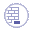Open the Bunny1.CATPart document.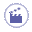1. Click Smooth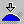.

 The Smooth dialog box is displayed.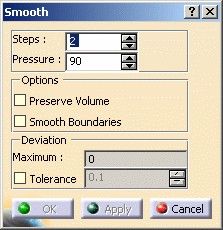2. Select the polygon.

3. Define the number of Steps of the smoothing between 1 and 100.

4. Define the Pressure value between 0 and 100.

 A value of 0 means there is no smoothing, and a value of 100 corresponds to the maximum smoothing.
5. Click Apply to preview the smoothing.

 The Maximum deviation is displayed.
6. Click OK.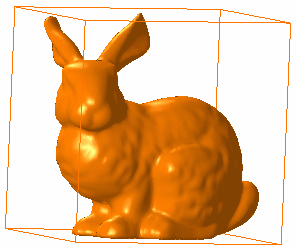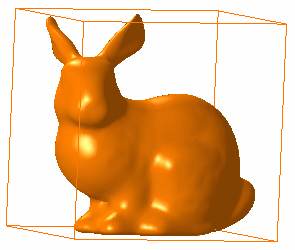The example above shows a smoothing using the following values: Steps = 1 Pressure = 10 The example above shows a smoothing using the following values: Steps = 1 Pressure = 90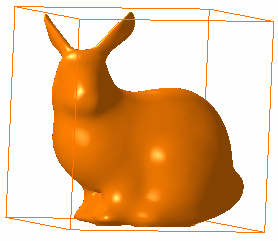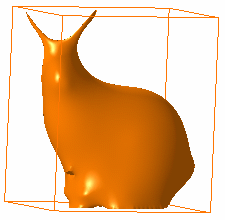The example above shows a smoothing using the following values: Steps = 40 Pressure = 10 The example above shows a smoothing using the following values: Steps = 40 Pressure = 90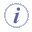The Preserve Volume option enables to keep the volume of the polygonal mesh after the smoothing (as you can see in the fourth example, the volume was not kept). The Smooth Boundaries option enables to smooth the boundaries (free edges) of the polygonal mesh. The Tolerance value enables to check if any of the vertices moved by a distance greater than the user specified tolerance. If the tolerance is violated, the vertices are restored to their original position and the smoothing is not performed. If successful, the Maximum Deviation of the vertices is displayed.